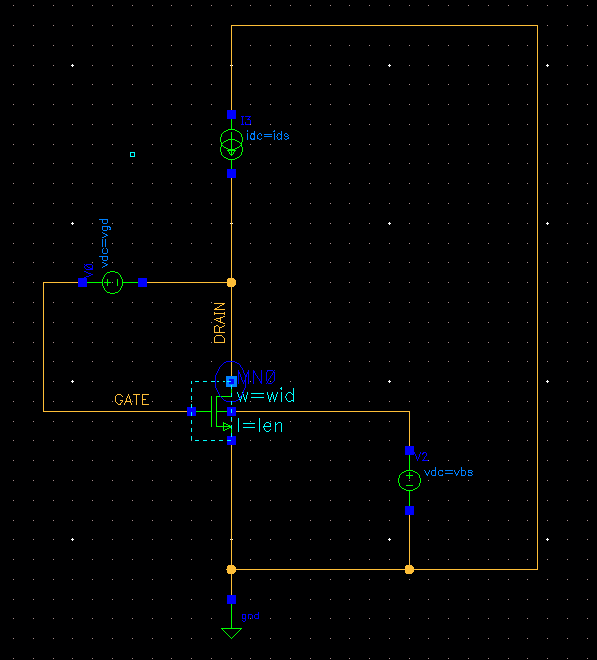# Setting up a MOS Characterization test bench in Cadence# Overview [top]

The MOS characterization test bench described here is the 1st thing I do when I am Getting Familiar with a Process. This setup allows me to get good idea about the device parameters as well as the matching. I use the data in my hand calculations to calculate the worst case headrooms, matching of mirrors and offsets of amplifiers.

# Schematic [top]

Create a schematic in Cadence that looks like the following:Notice here the current and the voltage values are setup as variables.

• We will run a DC sweep of the drain current and save the operating point for the whole sweep.
• Create a text file and add the following:
```save MN0:oppoint
```
Note here that MN0 should be a BSIM model device already. Sometimes it may be a subcircuit in that case MN0 should be replaced by MN0.mnbsim, etc.
• Now save this file somewhere and include it in the Setup->Simulation Files->Definition Files list so that it gets included in the netlist
• Now setup the variables as follows:
```wid = Any width you want to characterize
len = Any Length you want to characterize
vgd = Gate to drain voltage can be set to 0 or any value if you want to simulate near triode regions or on the other end hot carrier effects
vbs = can be set to the Bulk Source voltage desired
ids = di*(TMP+273)/300 - if the current is to be PTAT in biasing otherwise just set it equal to di
di = average current to characterize
IDL = current for lower extreme measurement = dil*(TMP+273)/300
IDH = current for higher extreme measurement = dih*(TMP+273)/300
dil = <val> here <val> is the lower tolerance of di. If not PTAT then set it simply to <val>
dih = <val> here <val> is the higher tolerance of di. If not PTAT then set it simply to <val>
TMP = temp (if PTAT operation) or 27 (If absolute values of dil and dih need to be used
```
• Now setup the DC sweep to sweep di from IDL to IDH.
• Now setup the measurements as:
```VTHL = value(v("NM0:vth" ?result "dc") VAR("IDL"))
VGSL = value(VS("/GATE") VAR("IDL"))
VDSATL = value(v("NM0:vdsat" ?result "dc") VAR("IDL"))
GML = value(getData("NM0:gm" ?result "dc") VAR("IDL"))
VTHH = value(v("NM0:vth" ?result "dc") VAR("IDH"))
VGSH = value(VS("/GATE") VAR("IDH"))
VDSATH = value(v("NM0:vdsat" ?result "dc") VAR("IDH"))
GMH = value(getData("NM0:gm" ?result "dc") VAR("IDH"))
ROL = (1 / value(getData("NM0:gds" ?result "dc") VAR("IDL")))
ROH = (1 / value(getData("NM0:gds" ?result "dc") VAR("IDH")))
IDSL = value(IS("/V4/PLUS") VAR("IDL"))
IDSH = value(IS("/V4/PLUS") VAR("IDH"))
```
• Now all the data can be generated for corners like typical and all skew with temperature to be imported in a chart like:```{
{
corners = "tttt",
temps = 27,
vars = "wid=4u len=10u di=1u dil=0.7u dih=1.3u TMP=temp",
},
{
corners = {"ffff", "ssss"},
temps = {27,125,-40},
vars = "wid=4u len=10u di=1u dil=0.7u dih=1.3u TMP=temp",
}
}
```